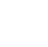# Introduction

While Loop is used to execute task multiple times until the specified condition is true

## Flow Chart

Syntax:

``` while(condition){ // code to be executed } ```

Explanation :

In place of Condition, you will write the condition until the task  should be executed

Now, Lets see some Examples :

## Example

Print  "Hello World"  20 times.

``` var i=1; while(i<=10){ console.log("Hello world"); i++; } //output: //Hello world //Hello world //Hello world //Hello world //Hello world //Hello world //Hello world //Hello world //Hello world //Hello world ```

Here,

We have declared a variable i=1

Next, We have used a while statement with a condition i<=10 Thatmeans, until and unless the value of i becomes 10 the console.log("Hello world"); and i++ will be executed.

We have used i++ to increment the value of i by 1 i.e. i=i+1

## Example

We will make a program that gives sum of first 10 natural numbers.

``` var sum = 0; var numbers = 10; var i=1; while(i <= numbers) { sum += i; i++; } console.log(sum); //output: //55 ```

Here,

We have declared variables sum=0 , number=0 and i=1 then we have used while loop to iterate i until its values becomes equal to numbers Every time value of i will Add to the Value of sum

Then, Value of sum is printed to the Console Tab.

Explanation: